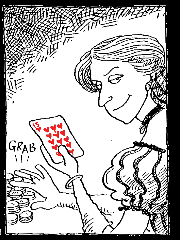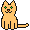Let Riverboat Lil READ YOUR MIND!

Here's what you do: Choose a random two digit number (e.g. 28). Add the two digits (2+8=10). Subtract the result from the number (28-10=18). Remember the result (18). Done that? Then CLICK HEREThis excellent game was programmed by Markus EichenbergerTricks and Games

The Murderous Maths main index page